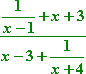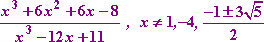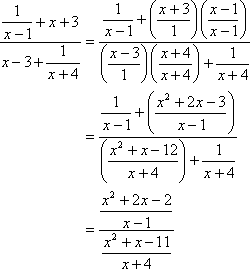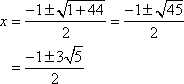Search
 Return to the Lessons Index  | Do the Lessons in Order  |  Print-friendly page

Complex Fractions: Technicalities

I started with this:

• Simplify the following expression:
•...and ended up with this:   Copyright © Elizabeth Stapel 2003-2011 All Rights ReservedThe first two domain restrictions were easy. The sub-fraction in the complex fraction's numerator will be undefined if x  1 = 0, and the sub-fraction in the denominator will be undefined if x + 4 = 0. The other restriction is harder. I had to consider the entire denominator. This would be zero when the numerator (of the denominator) was zero. Converting to a fraction, I got this:The denominator will be zero when x2 + x  11 is zero. Solving, I get:Return to the lesson

Top  |  Return to Index

Study Skills Survey

Tutoring from Purplemath
Find a local math tutor

 Copyright © 2021  Elizabeth Stapel   |   About   |   Terms of Use   |   Linking   |   Site Licensing Contact Us